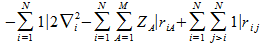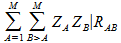# Quantumbiochemistry.org

## The adiabatic approximation : The motion of the nuclei and the electrons don't influence one another

The mass of nuclei is much higher than the mass of the electrons. As a result the motion of the electrons changes immediately when the nuclei change position but the nuclei will not adapt their position as fast when the electrons change their position. Consequently we assume the electronic wave-function only depends on the fixed positions of the nucleï.

We can thus split the molecular Hamilonian in an electronic part and a nuclear part:

The electronic hamiltonian is:.

The nuclear part is :

It is the contribution of the electrostatic foreces between the nucleï.The sum of these two terms is the hamitonian operator H in the equation Hψ = Eψ , and ψ and E are unknown.

This is also known as the Born-Oppenheimer approximation:

We only need to find a solution for the electronic part of the wave-function and the positions of the nuclei are chosen positions.

If our model represents a system in equilibrium then the nuclei have a position corresponding to their average position. The average nuclear position can be determined experimentally. This average position will most likely correspond to the ground-state.

When we think up a model we should use a set of nuclear positions that have some scientific justification. We can use the nuclear positions obtained from experiment. Alternatively we can try to calculate the nuclear positions that correspond to energy minima.